JMSLTM Numerical Library 7.2.0
com.imsl.stat

## Class GARCH

• All Implemented Interfaces:
Serializable, Cloneable

public class GARCH
extends Object
implements Serializable, Cloneable
Computes estimates of the parameters of a GARCH(p,q) model.

The Generalized Autoregressive Conditional Heteroskedastic (GARCH) model is defined as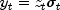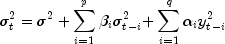where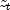's are independent and identically distributed standard normal random variables,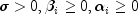and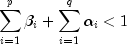The above model is denoted as GARCH(p, q). The \alpha_i, i=1,\ldots,q <\equation> and the \beta_j, j=1,\ldots,p <\equation> parameters will be called the ARCH and GARCH coefficients, respectively. When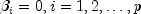, the above model reduces to ARCH(q) which was proposed by Engle (1982). That is, a GARCH(0,q) is equivalent to the ARCH(q) model. The non-negativity conditions on the parameters imply a nonnegative variance and the condition on the sum of the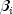's and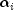's is required for wide sense stationarity.

In the empirical analysis of observed data, GARCH(1,1) or GARCH(1,2) models have often found to appropriately account for conditional heteroskedasticity (Palm 1996). This finding is similar to linear time series analysis based on ARMA models.

It is important to notice that for the above models positive and negative past values have a symmetric impact on the conditional variance. In practice, many series may have strong asymmetric influence on the conditional variance. To take into account this phenomena, Nelson (1991) put forward Exponential GARCH (EGARCH). Lai (1998) proposed and studied some properties of a general class of models that extended linear relationship of the conditional variance in ARCH and GARCH into nonlinear fashion.

The maximal likelihood method is used in estimating the parameters in GARCH(p,q). The log-likelihood of the model for the observed series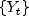with length m is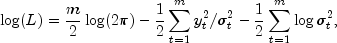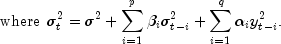In the model, if q = 0, the model GARCH is singular such that the estimated Hessian matrix H is singular.

The initial values of the parameter array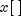entered in array xguess[ ] must satisfy certain constraints. The first element of xguess refers to sigma and must be greater than zero and less than maxSigma. The remaining p+q initial values must each be greater than or equal to zero but less than one.

To guarantee stationarity in model fitting,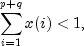is checked internally. The initial values should be selected from the values between zero and one. The value of Akaike Information Criterion is computed by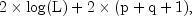where log(L) is the value of the log-likelihood function at the estimated parameters.

In fitting the optimal model, the class MinConGenLin, is modified to find the maximal likelihood estimates of the parameters in the model. Statistical inferences can be performed outside of the class GARCH based on the output of the log-likelihood function (getlogLikelihood method), the Akaike Information Criterion (getAkaike method), and the variance-covariance matrix (getVarCovarMatrix method).

Example, Serialized Form
• ### Nested Class Summary

Nested Classes
Modifier and Type Class and Description
static class  GARCH.ConstrInconsistentException
The equality constraints are inconsistent.
static class  GARCH.EqConstrInconsistentException
The equality constraints and the bounds on the variables are found to be inconsistent.
static class  GARCH.NoVectorXException
No vector X satisfies all of the constraints.
static class  GARCH.TooManyIterationsException
Number of function evaluations exceeded 1000.
static class  GARCH.VarsDeterminedException
The variables are determined by the equality constraints.
• ### Constructor Summary

Constructors
Constructor and Description
GARCH(int p, int q, double[] y, double[] xguess)
Constructor for GARCH.
• ### Method Summary

Methods
Modifier and Type Method and Description
void compute()
Computes estimates of the parameters of a GARCH(p,q) model.
double getAkaike()
Returns the value of Akaike Information Criterion evaluated at the estimated parameter array.
double[] getARCH()
Returns the estimated values of the ARCH coefficients.
double[] getGARCH()
Returns the estimated values of the GARCH coefficients.
double getLogLikelihood()
Returns the value of Log-likelihood function evaluated at the estimated parameter array.
double getSigma()
Returns the estimated value of sigma squared.
double[][] getVarCovarMatrix()
Returns the variance-covariance matrix.
double[] getX()
Returns the estimated parameter array, x.
void setMaxSigma(double maxSigma)
Sets the value of the upperbound on the first element (sigma) of the array of returned estimated coefficients.
• ### Methods inherited from class java.lang.Object

clone, equals, finalize, getClass, hashCode, notify, notifyAll, toString, wait, wait, wait
• ### Constructor Detail

• #### GARCH

public GARCH(int p,
int q,
double[] y,
double[] xguess)
Constructor for GARCH.
Parameters:
p - An int scalar containing the number of GARCH parameters.
q - An int scalar containing the number of ARCH parameters.
y - A double array containing the observed time series data.
xguess - A double array of length p + q + 1 containing the initial values for the parameter array.
Throws:
IllegalArgumentException - is thrown if the dimensions of y, and xguess are not consistent.
• ### Method Detail

• #### compute

public final void compute()
throws GARCH.ConstrInconsistentException,
GARCH.EqConstrInconsistentException,
GARCH.NoVectorXException,
GARCH.TooManyIterationsException,
GARCH.VarsDeterminedException
Computes estimates of the parameters of a GARCH(p,q) model.
Throws:
GARCH.ConstrInconsistentException - is thrown if the equality constraints are inconsistent.
GARCH.EqConstrInconsistentException - is thrown if the equality constraints and the bounds on the variables are found to be inconsistent.
GARCH.NoVectorXException - is thrown if no vector X satisfies all of the constraints.
GARCH.TooManyIterationsException - is thrown if the number of function evaluations exceeded 1000.
GARCH.VarsDeterminedException - is thrown if the variables are determined by the equality constraints.
• #### getAkaike

public double getAkaike()
Returns the value of Akaike Information Criterion evaluated at the estimated parameter array. Note that the compute method must be invoked first before invoking this method. Otherwise, the return value is 0.
Returns:
a double scalar containing the value of Akaike Information Criterion evaluated at the estimated parameter array.
• #### getARCH

public double[] getARCH()
Returns the estimated values of the ARCH coefficients. Note that the compute method must be invoked first before invoking this method. Otherwise, the method throws a NullPointerException exception.
Returns:
a double array of size q containing the estimated values of the moving average (ARCH) coefficients.
• #### getGARCH

public double[] getGARCH()
Returns the estimated values of the GARCH coefficients. Note that the compute method must be invoked first before invoking this method. Otherwise, the method throws a NullPointerException exception.
Returns:
a double array of size p containing the estimated values of autoregressive (GARCH) parameters.
• #### getLogLikelihood

public double getLogLikelihood()
Returns the value of Log-likelihood function evaluated at the estimated parameter array. Note that the compute method must be invoked first before invoking this method. Otherwise, the return value is 0.
Returns:
a double scalar containing the value of Log-likelihood function evaluated at the estimated parameter array.
• #### getSigma

public double getSigma()
Returns the estimated value of sigma squared. Note that the compute method must be invoked first before invoking this method. Otherwise, the return value is NaN.
Returns:
a double scalar containing the estimated value of sigma squared.
• #### getVarCovarMatrix

public double[][] getVarCovarMatrix()
Returns the variance-covariance matrix. Note that the compute method must be invoked first before invoking this method. Otherwise, the method throws a NullPointerException exception.
Returns:
a double matrix of size p + q + 1 by p + q + 1 containing the variance-covariance matrix.
• #### getX

public double[] getX()
Returns the estimated parameter array, x. Note that the compute method must be invoked first before invoking this method. Otherwise, the method throws a NullPointerException exception.
Returns:
a double array of size 1 + q + p containing the estimated values of sigma squared, the ARCH parameters, and the GARCH parameters.
• #### setMaxSigma

public void setMaxSigma(double maxSigma)
Sets the value of the upperbound on the first element (sigma) of the array of returned estimated coefficients.
Parameters:
maxSigma - A double scalar containing the value of the upperbound on the first element (sigma) of the array of returned estimated coefficients. Default = 10.
JMSLTM Numerical Library 7.2.0